# The Speed of Light - A Limit on Principle?

## A physicist's view on an old controversy

Last modification 13th October '97

by Laro Schatzer <schatzer@ubaclu.unibas.ch>

### "Easy" Treatise

Contemporary physics states that no object should be able to travel faster than the speed of light

c = 299'792'458 metres per second.

Although the value of c appears to be enormous when compared with conventional traveling speeds, it suggests a limit which renders a practical realization of interstellar travel improbable. Whereas another planet in our solar system is reachable within minutes or at least hours at the speed of light, a journey to the nearest star system Alpha Centauri would already demand a traveling time of several years. Surely, the question remains: Are faster-than-light speeds possible? At the present time most scientists believe that the correct answer should be "no". However, it has to be emphasized that there is no definite proof for this claim. Actually, whether superluminal speeds are possible in principle depends on the real structure of the space-time continuum, which contemporary physics ignores, however. Basically, there exist two distinct notions of space-time in physics, both of which represent a possibility:
• Galilean Space-Time (GST)
• Minkowski Space-Time (MST)
Briefly, whereas Galilean space-time allows the realization of faster-than-light speeds, at least in principle, Minkowski space-time does not. What is the reason for this difference? In the next sections it is exposed that the key point is the conception of global time, ie. the physical significance of the term simultaneity. In fact, what does it mean when we call two spatially separated events "simultaneous", actually? What we need is a clear physical notion of past, present and future, not only on a local but on a global level.

It is important to note that without some definition of global time the physical quantity speed (and thus light-speed) has no definite meaning anyway. Why? Consider an example: Imagine an object moving from position A to B. Its speed v is given by the formula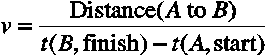Here, the start time t(A,start) and the finish time t(B,finish) are read off from two spatially separated clocks: one clock is located at point A and the other one at point B. Now, the difference of the two times in the denominator t(B,finish) - t(A,start) is an indefinite expression, unless there exists a rule how to synchronize both clocks, because clock B ignores the "current" time at clock A at first. But, in fact, the decision in favour of a particular synchronization rule is pure convention, because it seems impossible to send an "instantenous" (infinitely fast) message from A to B like "Initialize the clocks now!". Thus, the actual quantity of speed is conventional too, depending on the particular choice of the simultaneity definition.

The question concerning global time is also important in the context of different reference frames. What is a reference frame? A reference frame R is simply a coordinate system of some observer. (For instance, let us imagine a physicist experimenting in his laboratory.) The observer attaches to all physical events personal coordinates, ie. space coordinates x, y, z (where?) and a time coordinate t (when?). Another observer in his personal reference frame R' attaches to all physical events another (not necessarily equal) set of coordinates x', y', z' and t'. (Let us here imagine another physicist who is working in a train moving with constant velocity v with respect to the reference frame R.) While two events may appear simultaneous in reference frame R (happening at equal time t), does this still hold in reference frame R' (at equal time t')? And while the physical laws have a particular form in frame R, does one obtain the same formulas in frame R' also? The answer is given by a theory which relates the new coordinates x', y', z', t' to the old ones x, y, z, t. Essentially, this is what a theory of relativity is all about.

Remark: For a better understanding of the distinct space-time concepts it is fruitful to study a geometrical representation of space-time, the space-time diagram (see below). In this picture four-dimensional space-time is reduced to two dimensions. Instead of three space x, y, z and one time coordinate t, one uses only one space and the time coordinate, x and t, respectively. (Obviously, it is much more easier to draw and think in two than in four dimensions.) For reasons of convenience the units are chosen such that the speed of light equals unity c=1. Hence, a light ray, which is described by x=+ct or x=-ct, appears as a straight line in the (x,t)-plane at 45° or 135°, respectively.

The reader is encouraged to reconstruct the arguments by studying the space-time diagram. Remember that the x-axis is the line of simultaneity (ie. with constant time t=0), and that the t-axis is the line of constant position (x=0).

#### Galilean Space-Time

In Galilean Space-Time the physical existence of an absolute time is assumed. The pioneer of physics Isaac Newton defined it in the following way :
"Absolute, true and mathematical time, in itself, and from its own nature, flows equally, without relation to any thing external; and by other name called Duration. Relative, apparent, and vulgar time, is some sensible and external measure of duration by motion, whether accurate or unequable, which is commonly used instead of true time; as an hour, a day, a month, a year. It may be, that there is no equable motion, whereby time may be accurately measured. All motions may be accelerated and retarded, but the flowing of absolute time is liable to no change."
Because of this absolute time the global notion of past, present and future is the same in all reference frames. If two events are simultaneous in one particular reference frame, this means that they are also simultaneous in all reference frames. Thus, there is a unique separation between past and future events - the line of present in the space-time diagram (see below). Within the framework of Galilean Space-Time, faster-than-light speeds are possible in principle. However, electromagnetical waves are limited not to exceed the speed of light c, which usually depends on the direction of the light signal the reference frame in which it is measured. The speed of light is constant only in the absolute space-time frame, which is also called the Newtonian rest frame.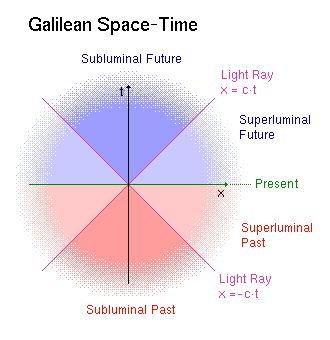There has been a variety of attempts to describe electromagnetical waves (light) as excitations of some medium, quite in analogy to sonic waves which propagate in the medium air. The hypothetical light medium was called the ether and it was supposed to be in rest in the absolute space-time frame. (That is why the absolute frame is also called ether frame sometimes.) Since the establishment of the theory of special relativity it has become extremely unpopular among scientists to speak about an "ether". But it is well known that electromagnetical waves can be indeed interpreted as excitations of some "medium". However, this medium is not a solid or a liquid in the sense of classical physics, but it is governed by the laws of quantum mechanics. In quantum field theory it is simply called vacuum ("void"). Some physicists prefer to interprete the vacuum as space-time itself, but this does not cover the fact that its true nature still remains a mystery. Anyhow, the term quantum ether might be used to indicate a thinkable modern synthesis of both concepts.

#### Minkowski Space-Time

Minkowski Space-Time does not know any absolute time which is physically meaningful. It was the revolutionary idea of Albert Einstein to give the notion of simultaneity a new definition. Especially, because all experimental tests to determine the motion with respect to some absolute space-time frame had failed, he decided to abandon the notion of absolute time at all. In the famous theory of relativity he postulated two principles which should hold for all physics:
1) All physical laws appear according to the same laws in all reference frames.
2) The speed of light is constant in all reference frames.
Now, while the first postulate seems well established by observation and experiments, the second one is simply an assumption. It implies, in contrast to Galilean Space-Time, that simultaneity is not an absolute physical quality, but a relative one, depending on the motion of the observer (ie. the reference frame). However, it has to be emphasized that although the existence of a physical absolute time (or, equivalently, a preferred reference frame) could not be established by experiments, the theory of special relativity does not disprove it either.

Now, how does the theory of relativity allow to compare the relative time of two events at distant positions? How can one synchronize clocks being spatially separated? The definition Albert Einstein offered, which is completely equivalent to his second postulate, is the following:

Choose two clocks (let us label them 1 and 2) in some reference frame R. In order to synchronize them place a mirror at position 2, then emit a light signal from clock 1 at space-time point A. The light signal arrives at clock 2 at space-time point B, it is reflected in the opposite direction and arrives at clock 1 at space-time point C (see the space-time diagram below). Since the speed of light is per definition constant and the light signal travels the same distance in both directions, the instant t(B) of the reflection equals exactly t(P), which is in the mean-time of A and C. Or, more formally, t(B) = t(P) = (t(A)+t(C))/2.
With this definition of global time, simultaneous events in one particular reference frame need not to be simultaneous in another frame. This can be checked by following the same procedure in a frame R' where all clocks are moving with relative speed v with respect to the former reference frame R.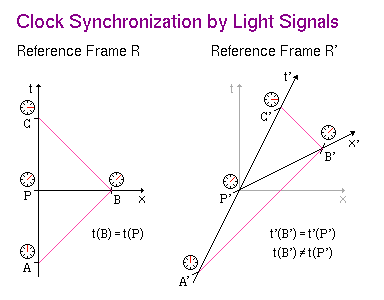Now, because absolute time and thus the Newtonian reference frame have disappeared in the theory of special relativity, all reference frames are completely equivalent. This implies that two superluminally separated events in space-time can be made instantenous by choosing a particular reference frame. Hence, present appears no more as a simple line in the space-time diagram, but it equals the whole region of "faster-than-light" processes. Furthermore, since there is no absolute reference frame separating the regions of superluminal past and future, faster-than-light motion in Minkowski space-time implies the possibility of time travel. Therefore, because this leads to the well known severe logical paradoxes of time travel, the theory of special relativity excludes faster-than-light speeds a priori.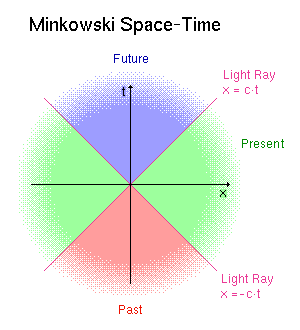#### Summary

The question whether the speed of light is a true physical limit has no definite answer yet. It depends on the real structure of the space-time continuum, which is presently unknown. If absolute time (and a preferred reference frame) exist, then faster-than-light speeds - and even faster-than-light travel - are possible, at least in principle. Although the theory of special relativity states against absolute time and superluminal phenomena, it does it not by proof, but only by assumption. If superluminal signals are to be discovered in the future, then the notion absolute time will surely have to be reintroduced to physics.

Are there indications that absolute time and faster-than-light processes exist? The opinion of the author is "yes"! It is the task of the next section to present some physical evidence.

### Physical Treatise

For the description of physical phenomena it is sufficient to use only the first of Einstein's postulates . Without loss of generality one may choose a reference frame R (with coordinates x, y, z, t) where the speed of light c is constant in all directions. The general coordinate transformation from this particular reference frame R to a general one R' (primed coordinates) reads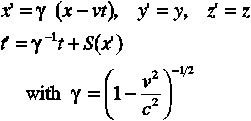where the relative speed v of R' with respect to R is chosen to be parallel to the x-axis. The transformation properly expresses the apparent contraction of moving rods (Lorentz-Fitzgerald contraction) and the slowing of moving clocks (time dilation). The function S(x') simply determines the notion of simultaneity in frame R'. Generally, S(x') can be an arbitrary function, but it is convenient to impose S(0) = 0 such that the clocks of the reference frames R and R' are synchronized at the origin (x,t) = (0,0) = (x',t'). Furthermore, in order to avoid acceleratory effects, one usually imposes that S(x') is linear in x', ie. S(x') = s x'.

#### Minkowski Space-Time

It can be shown that Einstein's second postulate is equivalent to setting S(x') = - v/c^2 x', so that one obtains the well known Lorentz transformation equations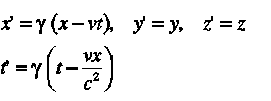with the speed of light c' = dr'/dt'(r=ct) = c constant in all frames. Thus, from the viewpoint of relativity, all reference frames are completely equivalent.

The first postulate ensures that physical phenomena have the same appearance in all reference frames, in the sense that one obtains the same result for all measurable quantities being but mean round-trip quantities (eg. the mean two-way speed of light). The second postulate states that there is no preferred reference frame and thus the physical laws (when expressed in formulas using global coordinates) appear equally in all reference frames. The space-time coordinates (Lorentz coordinates) are defined in such a way that the one-way speed of light is constant.

The success of the theory of relativity can be understood from the fact that the possibility to formulate all physical laws covariantly, ie. in a relativistically invariant manner, appears most tempting. One cannot deny that the involved mathematics is highly attractive from an esthetical point of view. For more information on special relativity and the principle of covariance one may consult eg. , .

#### Galilean Space-Time

Another possibility is to set S(x') = 0 leading to the affine coordinate transformation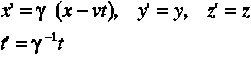It has to be emphasized that these equations are not equivalent although similar to the well-known transformation equations of Galilean relativity,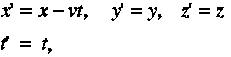as the former equations contain additional time dilation and length contraction factors expressing the Lorentz-Fitzgerald contraction hypothesis.

In the Galilean framework the reference frame R (with unprimed coordinates x, t) has a special significance: It is the Newtonian frame of absolute time and space.

Although the one-way speed of light is not constant in general (ie. when expressed in an arbitrary reference frame), the mean-speed c of a round-trip is again constant , what is in accordance with all experiments (like Michelson-Morley a.s.o.). It should be emphasized again that there has been no experiment which determined the one-way speed of light , since this would require the possibility of synchronizing physical clocks by some other means than finite-speed signals. Thus, in fact, some "experimental proof" of the constancy of the one-way speed of light has not been given so far.

Remark: It has to be noted that H. A. Lorentz version of the ether theory (which is set in such a Newtonian framework), ie. Lorentz relativity, is a valid alternative to special relativity. It suffices to introduce the hypothesis that moving particles are contracted by some interaction with the ether (Lorentz-FitzGerald contraction), and that internal time is dilated by the same factor.

#### Towards a Decision

Which conception of space-time structure is the physically correct one? Obviously, the covariant framework is the most attractive one to describe matter in electromagnetical and gravitational fields. However, it is still possible that there exists an underlying absolute time preserving causality for superluminal phenomena. The theory of relativity does not offers an adequate framework for superluminal processes, at least not without refering to logical paradoxes, but a Galilean theory does. As is pointed out in the following section, several arguments can be found which indicate the non-generality of covariance and the existence of superluminal processes. The resurrection of absolute time in physics is therefore possible, if not even necessary.

#### The Non-Generality of Covariance

Besides the principle of relativity, quantum mechanics is a cornerstone of modern physics. No physical theory evades relativity and quantum mechanics, but do these cornerstones actually fit together? Let us repeat what is the time evolution of a physical state |s> in quantum mechanics (according to the Copenhagen interpretation). There are two steps:
1) The unitary time evolution |s(t)> = U(t) |s(0)>
2) The reduction of the state |s(t)> into an eigenstate of an observable P |s(t)> in case of measurement by an observer, where P is a projection operator. This is the famous "collapse of the wavefunction".
The unitary time evolution is represented covariantly in a natural way, for instance, it leads to the Klein-Gordon or Dirac equation in the case of a relativistic particle. However - and what is less well known - there exists no covariant representation of the state reduction postulate . If a physical reality is attached to the wave function, then the theory of relativity fails bitterly. In this context also belong EPR-like effects , which imply miraculous non-local (superluminal) correlations of measured quantities. Albert Einstein and other physicists could not believe in the validity of quantum mechanics because of such effects, which are apparently in conflict with the theory of relativity. One example is the violation of the Bell inequalities , which has been confirmed experimentally . Thus, quantum mechanics has proven to be correct (see  for an overview). Although non-local effects are a constituent of quantum mechanics, most physicists still believe in the validity of special relativity, because EPR-like effects have not allowed to transmit information at superluminal speeds so far. Yet, EPR-correlations remain a mystery if local realism is assumed to be valid. Therefore, the possibility of superluminal communication (and thus FTL travel) has been acknowledged by various authors, eg. .

While time and space appear somehow "on equal rights" in the Lorentz transformation equations, this is not the case within the formalism of quantum mechanics. In the quantum field equations the position of a particle is described by a linear operator (a hermitian operator) in the Hilbert space of physical states, whereas the time coordinate appears as an exterior parameter only. It is well known that it is impossible to construct a valid time operator. There exist no time eigenstates, what is basically a consequence of Heisenberg's uncertainity relation of energy-time. Therefore, there exists no covariant 4-position operator in quantum mechanics. This is one of the main reasons why it has not yet been possible to construct a reasonable quantum field theory of gravitation. Thus, it is evident that the standard theory of relativity and quantum mechanics are incompatible.

#### Some Arguments in Favour of Absolute Time

One possible solution to the problem of time in quantum mechanics (and thus in quantum gravity) would be the reintroduction of a background Newtonian time. There are serious attempts to quantize gravitation in such a framework, eg. Post-Relativistic Gravity. This solution is also considered in more advanced research programs, eg. Canonical Quantum Gravity (see section "Further reading").

Moreover, there are some heuristic arguments which might further motivate the reintroduction of absolute time:

First, if there is a physical absolute time, then the number of fundamental constants reduced by one, since the (one-way) speed of light is not a constant any longer. This leads to a simplification and a new interpretation of the physical quantities and constants .

Second, it is well known that one can define a universal time, which appears in cosmological models. For instance, general relativity leads one to the Robertson-Walker metric , which describes the long-range structure of our universe: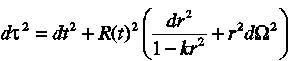Here, the time parameter t defines an universal time, the cosmological time. If there was an absolute beginning (with the big bang), it can be identified with the age of the universe. Anyhow, adopting absolute time would give it a further physical meaning. And, of course, there exists a measurable preferred reference frame, which can be determined, for instance, from the absolute motion towards the uniform cosmic background radiation.

Interestingly, recent investigations of electromagnetic radiation propagating over cosmological distances seem to reveal a true anisotropy in the structure of our universe, suggesting that the speed of light might be not a true constant, but dependent on direction and polarization. These results might possibly represent a further indication in favour of the existence of an absolute reference frame .

#### Summary

Which is the real space-time structure? Both Galilean space-time and Minkowski space-time have appeared to be valid physical concepts. However, the absolute generality of relativistic covariance is set into doubt by the following arguments:
• The time evolution of a quantum mechanical state has no covariant representation, because the "measurement process" cannot be described in a relativistically invariant manner.
• EPR-like effects seem to indicate non-local (superluminal) processes.
• It is impossible to construct a quantum time observable, so that no covariant 4-position operator exists.
• From a cosmological perspective the existence of a preferred reference frame appears to be natural.
It has been argued that a solution to these incompatibilities could be the reintroduction of absolute time to physics. Thus, the concept of Galilean Space-Time might be the correct one after all. Incidentally, there are active research groups trying to experimentally detect the existence of a preferred reference frame in this context.

Conclusion: If our universe has a Newtonian background, ie. if there is an absolute time underlying the space-time continuum, then there is no threat on causality by superluminal processes, because time travel and its paradoxes are excluded a priori. And thus, within this framework, faster-than-light travel is possible, at least in principle.

Remark: It may be a surprise for many physicists that even within the framework of general relativity faster-than-light speed is allowed, provided that the space-time metric of the universe is globally hyperbolic . This condition simply implies that closed time-like paths in space-time (and thus time-travel) are excluded, so that causality is again preserved. (In this framework, the cosmological time parameter can be again interpreted as the absolute time of the universe. However, in order to construct a propulsion mechanism for faster-than-light travel, exotic matter (with imaginary mass) would probably be needed in order to produce negative energy densities in space. Unfortunately, exotic matter is not known to exist, although negative energy densities have been shown to appear in quantum field theory. But, of course, such a hypothetical propulsion mechanism just provokes to be given the familiar name of the warp drive.

### References

 I. Newton: "Mathematical Principles of natural philosophy", (London, Dawson, 1969)
 J. P. Hsu, L. Hsu: "A physical theory based solely on the first postulate of relativity", Physics Letters A 196 (1994), pgs. 1-6; F. Selleri: "Theories equivalent to special relativity", in Frontiers of Fundamental Physics, edited by M. Barone and F. Selleri, (Plenum Press, New York, 1994)
 H. Reichenbach: "The philosophy of space and time", (Dover, New York, 1958)
 J. D. Jackson: "Classical electrodynamics", (Wiley, New York, 1975), chapter 11
 Y. Aharonov, D. Z. Albert: "Can we make sense of the measurement process in relativistic quantum mechanics?", Physical Review D 24 (1981), pgs. 359-370; A. Peres: "Relativistic Quantum Measurements", Annals of the New York Academy of Sciences, Volume 755 (1995) ("Fundamental Problems in Quantum Theory"), pgs. 445-450
 A. Einstein, B. Podolsky, N. Rosen: "Can quantum-mechanical description of physical reality be considered complete?", Physical Review 47 (1935), pp. 777
 J. S. Bell: "On the Einstein Podolsky Rosen paradox", Physics 1 (1964), No. 3, pp. 195
 A. Aspect et al.: "Experimental realization of Einstein-Podolsky-Rosen-Bohm gedankenexperiment: A new violation of Bell's inequalities", Physical Review Letters 49 (1982), No. 2, p. 91; "Experimental test of Bell's inequalities using time-varying analyzers", Physical Review Letters 49 (1982), No. 25, pp. 1804
 R. Y. Chiao, P. G. Kwiat, A. M. Steinberg: "Faster than light?", in Scientific American (1993), August
 O. Steinmann: "The EPR Bingo", Helv. Phys. Acta, Vol. 69 (1996), pgs. 702-705
 S. Weinberg: "Gravitation and cosmology", (Wiley, New York, 1972), chapter 14
 B. Nodland, J. P. Ralston: "Indication of Anisotropy in Electromagnetic Propagation over Cosmological Distances", Physical Review Letters 78 (1997), No. 16. 3043-3046; e-print:astro-ph/9704196; see also here
 M. Alcubierre: "The warp drive: hyper-fast travel within general relativity". Classical and Quantum Gravity 11 (1994), pgs. L73-L77, see also here.

• C. J. Isham: "Prima Facie Questions in Quantum Gravity": Relativity, Classical and Quantum, eds. J. Ehlers and H. Friedrich, Springer-Verlag, Berlin (1994), e-print:gr-qc/9310031
• G. K. Au: "The Quest for Quantum Gravity", e-print:gr-qc/9506001

### Related Pages on the Web

#### "Grand Unified Theories":

• Brian Greene: "Superstring Theory". Superstring theory appears to be a very promising attempt to unite all fundamental forces including gravity, but it is also not able to resolve the measurement problem. However, it resides on a fixed space-time background, and it does allow the existence of a background time parameter.